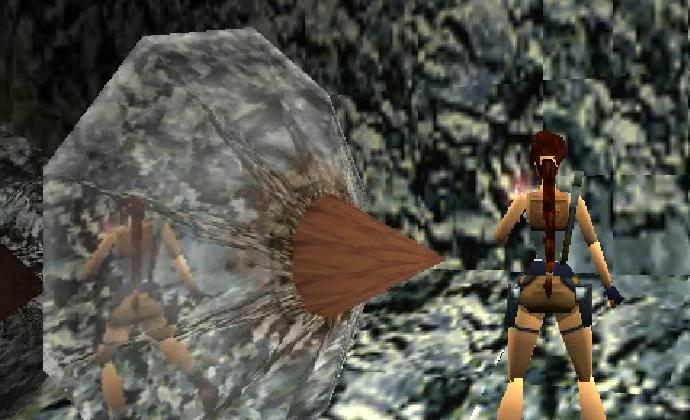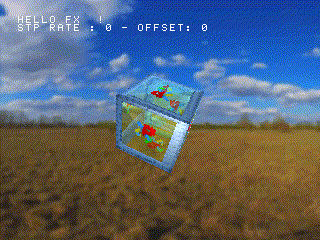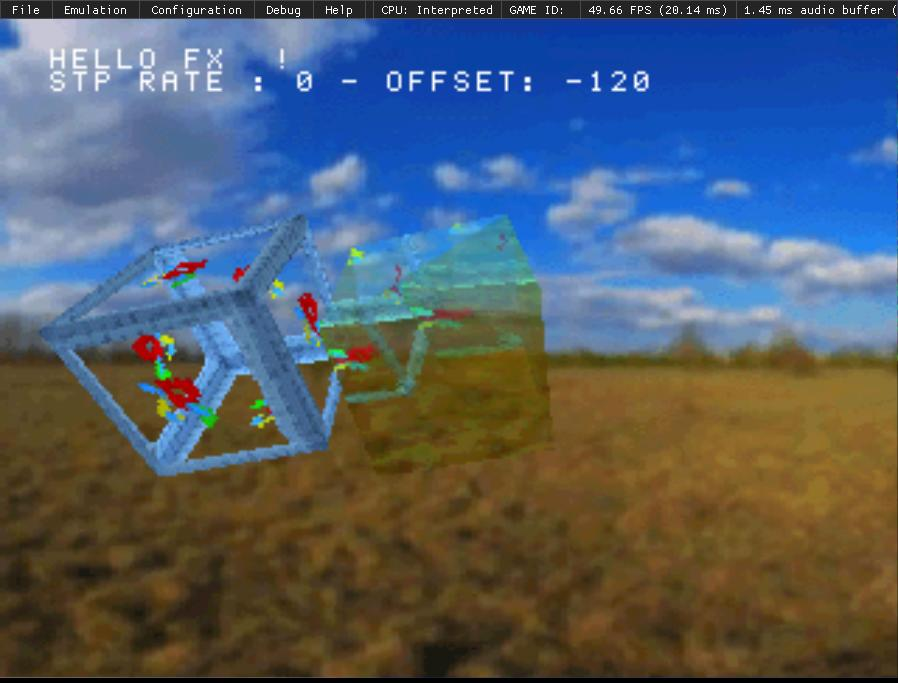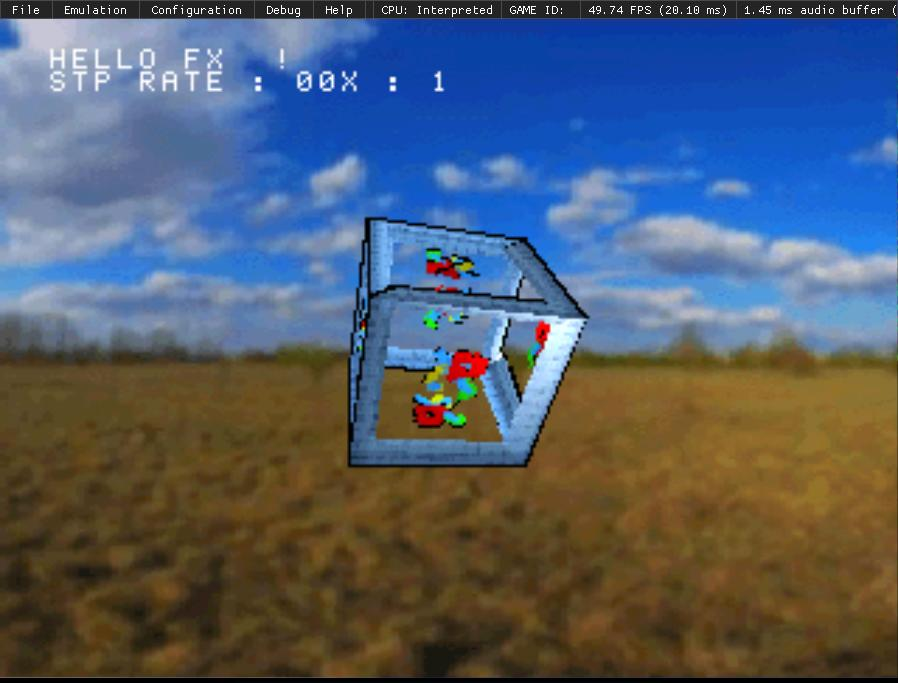# RE: Pseudo-reflections in TRII on Psx - the code part II

Following up on my follow-up to an article about a pseudo-reflection effect in the Tomb Raider I & II games on psx, picking up where we left of ;

In this pic, you can see that Lara's reflection looks cloudy. This could be because a second primitive with semi-transparency is drawn above the one doing the reflection.Now this might sound a bit expensive to draw two primitives instead of one, but it's not really. In fact, you can do the calculations once, and apply the resulting coordinates to 2 different primitives.

``````// cast nextpri as POLY_GT3
poly = (POLY_GT3 *)nextpri;
poly = (POLY_GT3 *)nextpri+sizeof(POLY_GT3);
// Initialize the primitives
SetPolyGT3(poly);
SetPolyGT3(poly);

// Reflection Cube
// This cube has its UVs mapped directly to VRAM coordinates
// We're using the framebuffers as a texture (0,0 and 0,256)
// Get 256x256 texture page that's at x0, y0
poly->tpage = getTPage( 2, stpRate,
0,
!(db) * 256  // Here, we're using db's value that can be either 0 or 1 to determine the texture page Y coordinate.
);
// Set STP
SetSemiTrans(poly, stpFlag);
// Map coordinates from drawarea (320x240) to texture size (256x240) in fixed point math
// x = x * (256 / 320) => ( x * ( 256 * 4096 ) / 320 ) / 4096 => x * 3276 >> 12
// y = y * (240 / 240) => ( y * ( 240 * 4096 ) / 240 ) / 4096 => y * 4096 >> 12  == y

setUV3( poly,
(poly->x0 * normH) >> 12,
poly->y0 - (!(db) * 16) , // We're using db's value again to add a 16 pixels offset to the Y's coordinates of the UVs
(poly->x1 * normH) >> 12,
poly->y1 - (!(db) * 16), // We have to do that because the buffer is 240 high, whereas our texture page is 256, hence 256 - 240 == 16
(poly->x2 * normH) >> 12,
poly->y2 - (!(db) * 16)
);

// Draw "container" cube
// This cube has a texture with transparent areas.
// STP bit is set on PNG's alpha channel : img2tim -usealpha -org 320 0 -o cube.tim cube.png
poly->tpage = getTPage( timImages.mode&0x3, stpRate,
timImages.prect->x,
timImages.prect->y
);

// If 8/4bpp, load CLUT to vram
if ( (timImages.mode & 0x3) < 2 ) {
setClut( poly,
timImages.crect->x,
timImages.crect->y
);
}
// Set UV coordinates
setUV3(poly, modelCube.u[i].vx, modelCube.u[i].vy,
modelCube.u[i+2].vx, modelCube.u[i+2].vy,
modelCube.u[i+1].vx, modelCube.u[i+1].vy
);
// Rotate, translate, and project the vectors and output the results into a primitive
// curTriangle, +1, +2 point to the vertices index of the triangle we're drawing.
OTz  = RotTransPers(&modelCube_mesh[ modelCube_index[ curTriangle ] ]  , ( long * ) &poly->x0, &p, &Flag);
OTz += RotTransPers(&modelCube_mesh[ modelCube_index[ curTriangle + 2] ], ( long*) &poly->x1, &p, &Flag);
OTz += RotTransPers(&modelCube_mesh[ modelCube_index[ curTriangle + 1] ], ( long * ) &poly->x2, &p, &Flag);

// Instead of doing the same calculations for the "prism" cube, use results from above directly
poly->x0 = poly->x0;
poly->y0 = poly->y0;
poly->x1 = poly->x1;
poly->y1 = poly->y1;
poly->x2 = poly->x2;
poly->y2 = poly->y2;``````

And there you have it :Here's a pic that hopefully shows a bit more :You can see the two cubes with the right one being the "reflection"...

You can easily get various graphical result by tweaking the colors/depth/size of the primitives !